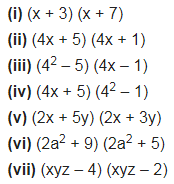# Use the identity (x + a) (x + b) = x2 + (a + b)x + ab to find the following products. (i) (x + 3) (x + 7)

Use the identity (x + a) (x + b) = x2 + (a + b)x + ab to find the following products.